## How to sum diagonal of a range in Excel?

When you do some arithmetic calculations, you may need to sum numbers diagonally in a table. In fact, you can also sum diagonal numbers in Excel which doesn’t require you to sum the numbers one by one. If you want to know more about this question, please read the following article.

Sum diagonal cells of a range in Excel

####Sum diagonal cells of a range in Excel

Supposing I have the following data range, and now I want to get the result of the summation of the diagonal cells.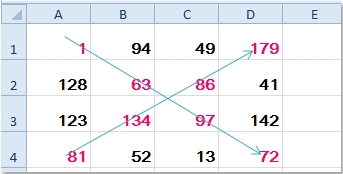1. Sum cells diagonal down and to the right

If you want to sum the cells diagonal from top left corner to lower right corner（A1+B2+C3+D4）, the following formulas can help you.

Please enter this formula =SUM(A1:D4*(ROW(A1:D4)=COLUMN(A1:D4))) into a blank cell, see screenshot: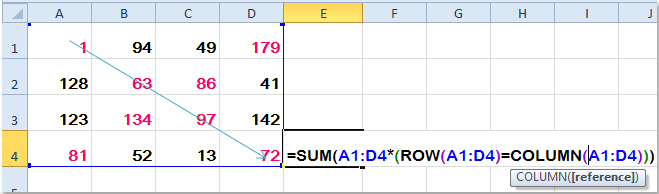And then press Ctrl + Shift + Enter keys together, and you will get the result as you want.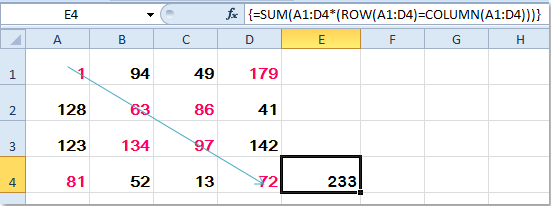2. Sum cells diagonal up and to the right

To sum the diagonal cells from lower left corner to top right corner (A4+B3+C2+D1), you should apply the following formula: =SUM(A1:D4*(ROWS(A1:D4)+1-ROW(A1:D4)=COLUMN(A1:D4))), and press Ctrl + Shift + Enter keys to get the correct result. See screenshots: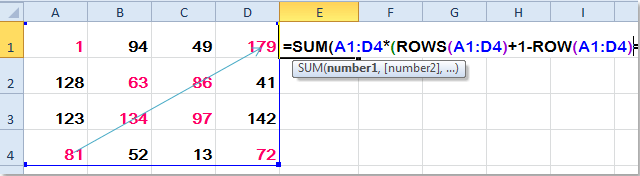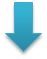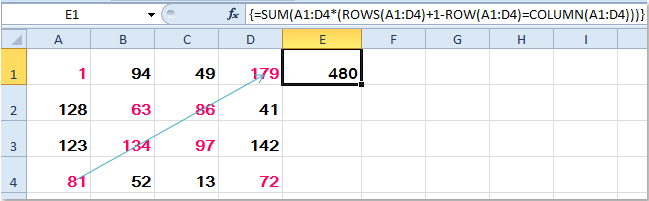Notes:

1. The arguments A1: D4 is the data range that you want to sum the diagonal cells.

2. These formulas only are applied to the range cells which have the same column number and row number, such as 5 column and 5 row.

Related article:

How to sum all digits in a number in Excel?

### Best Office Productivity Tools

 Popular Features: Find, Highlight or Identify Duplicates   |  Delete Blank Rows   |  Combine Columns or Cells without Losing Data   |   Round without Formula ... Super Lookup: Multiple Criteria VLookup  |   Multiple Value VLookup  |   VLookup Across Multiple Sheets   |   Fuzzy Lookup .... Advanced Drop-down List: Quickly Create Drop Down List   |  Dependent Drop Down List   |  Multi-select Drop Down List .... Column Manager: Add a Specific Number of Columns  |  Move Columns  |  Toggle Visibility Status of Hidden Columns  |  Compare Ranges & Columns ... Featured Features: Grid Focus   |  Design View   |   Big Formula Bar   |  Workbook & Sheet Manager   |  Resource Library (Auto Text)   |  Date Picker   |  Combine Worksheets   |  Encrypt/Decrypt Cells   |  Send Emails by List   |  Super Filter   |   Special Filter (filter bold/italic/strikethrough...) ... Top 15 Toolsets:  12 Text Tools (Add Text, Remove Characters, ...)   |   50+ Chart Types (Gantt Chart, ...)   |   40+ Practical Formulas (Calculate age based on birthday, ...)   |   19 Insertion Tools (Insert QR Code, Insert Picture from Path, ...)   |   12 Conversion Tools (Numbers to Words, Currency Conversion, ...)   |   7 Merge & Split Tools (Advanced Combine Rows, Split Cells, ...)   |   ... and more

Supercharge Your Excel Skills with Kutools for Excel, and Experience Efficiency Like Never Before. Kutools for Excel Offers Over 300 Advanced Features to Boost Productivity and Save Time.  Click Here to Get The Feature You Need The Most...#### Office Tab Brings Tabbed interface to Office, and Make Your Work Much Easier

• Enable tabbed editing and reading in Word, Excel, PowerPoint, Publisher, Access, Visio and Project.
• Open and create multiple documents in new tabs of the same window, rather than in new windows.
• Increases your productivity by 50%, and reduces hundreds of mouse clicks for you every day!No ratings yet. Be the first to rate!
This comment was minimized by the moderator on the site
Thanks.What formula do i use to sum everything to right or the left of the diagonal?
This comment was minimized by the moderator on the site
Thanks for finally writing about >How to sum diagonal of a range in Excel?
This comment was minimized by the moderator on the site
Thank you so much for your good information, I would like to know how I can multiply a triangular range say a triangle SUM of triangle A1,E1,A5, (this triangle is static and used as the "multiplier"). So this range of cells multiplies the range of cells G1,K1,G5 then the results are put in the range of cells I1,M1,I5. Once this is done I want to be able to slide down the triangle G1 because G2, K1 becomes K2 and G5 becomes G6. and so on with all the results put in the triangle where I1 become I2 and so on. Thank you so much for your help
This comment was minimized by the moderator on the site
This is great! how can I SUM the triangle in figure 2? Imagine a triangle that has the following 3 corners A1 D1 A4 thank you so much!!!
This comment was minimized by the moderator on the site
Hi there, What would the formula be if I wanted for 2nd scenario if the matrix started on, say, D22? In other words, how do I compensate for larger row/column numbers than starting at 1 (ie A1)? Thanks, Karl
This comment was minimized by the moderator on the site
Instead of ROW(A1:D4) = COLUMN(A1:D4), use: (ROW(A1:D4)-COLUMN(A1:D4))=(ROW()-COLUMN())
There are no comments posted here yet